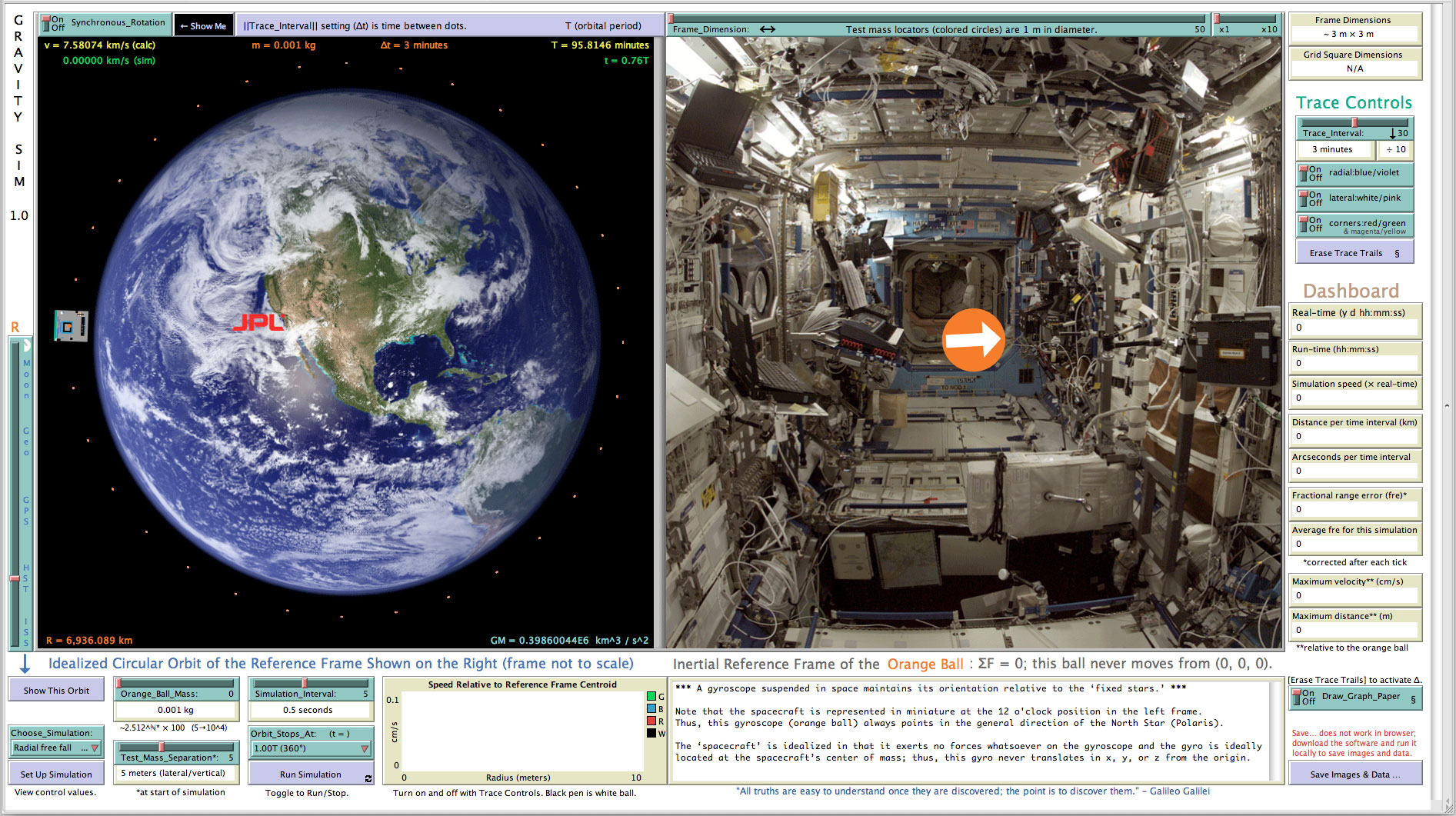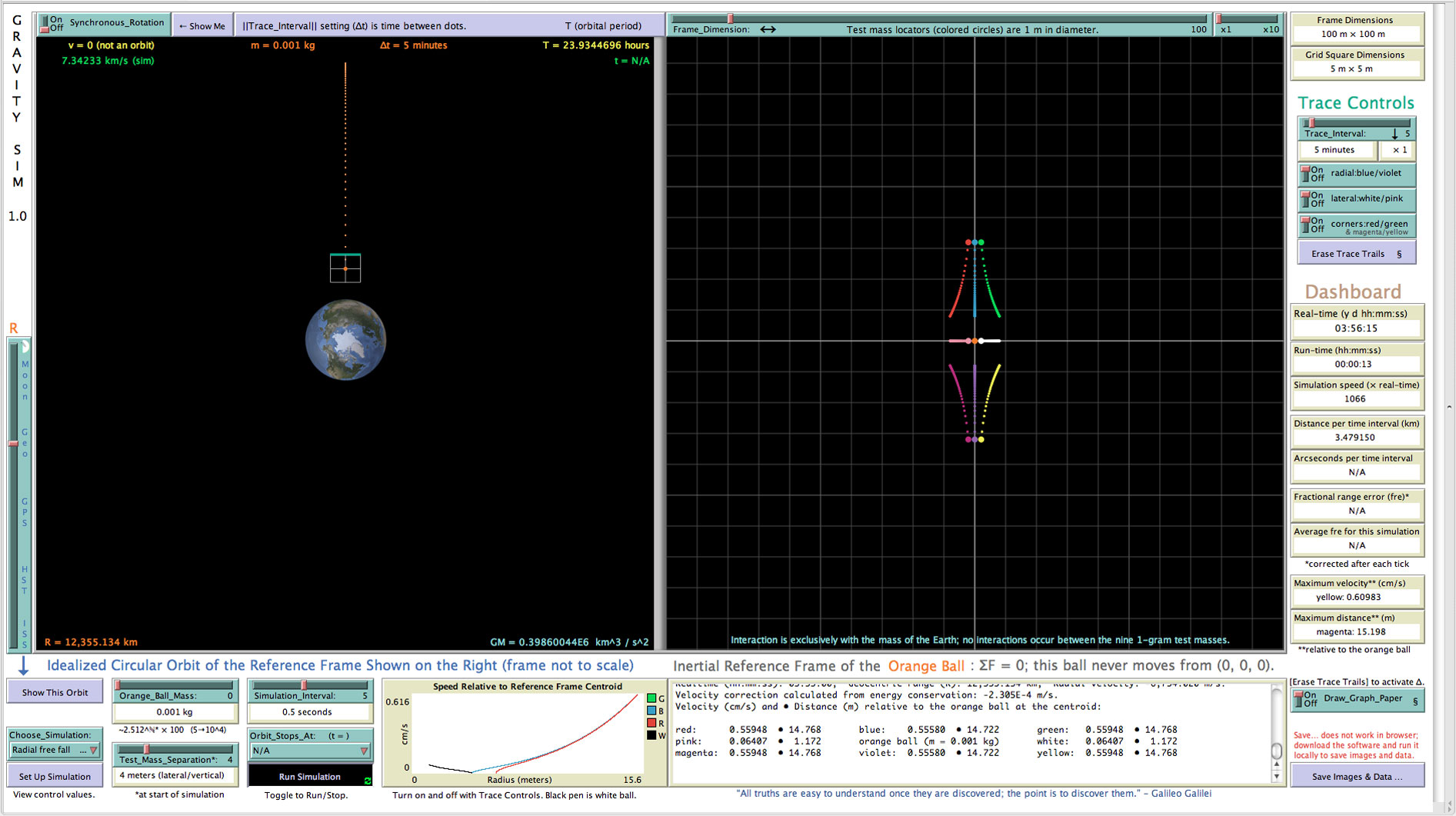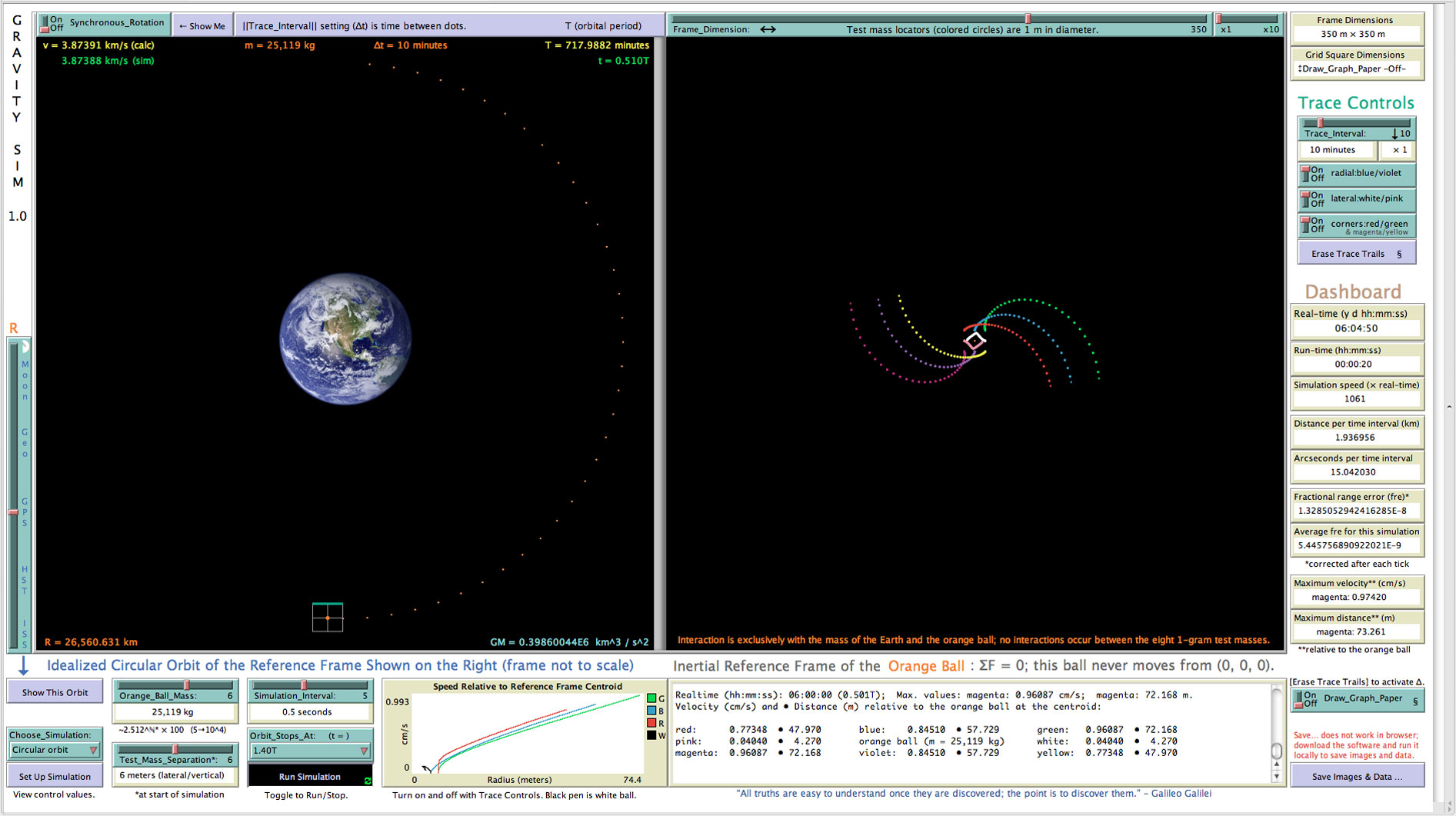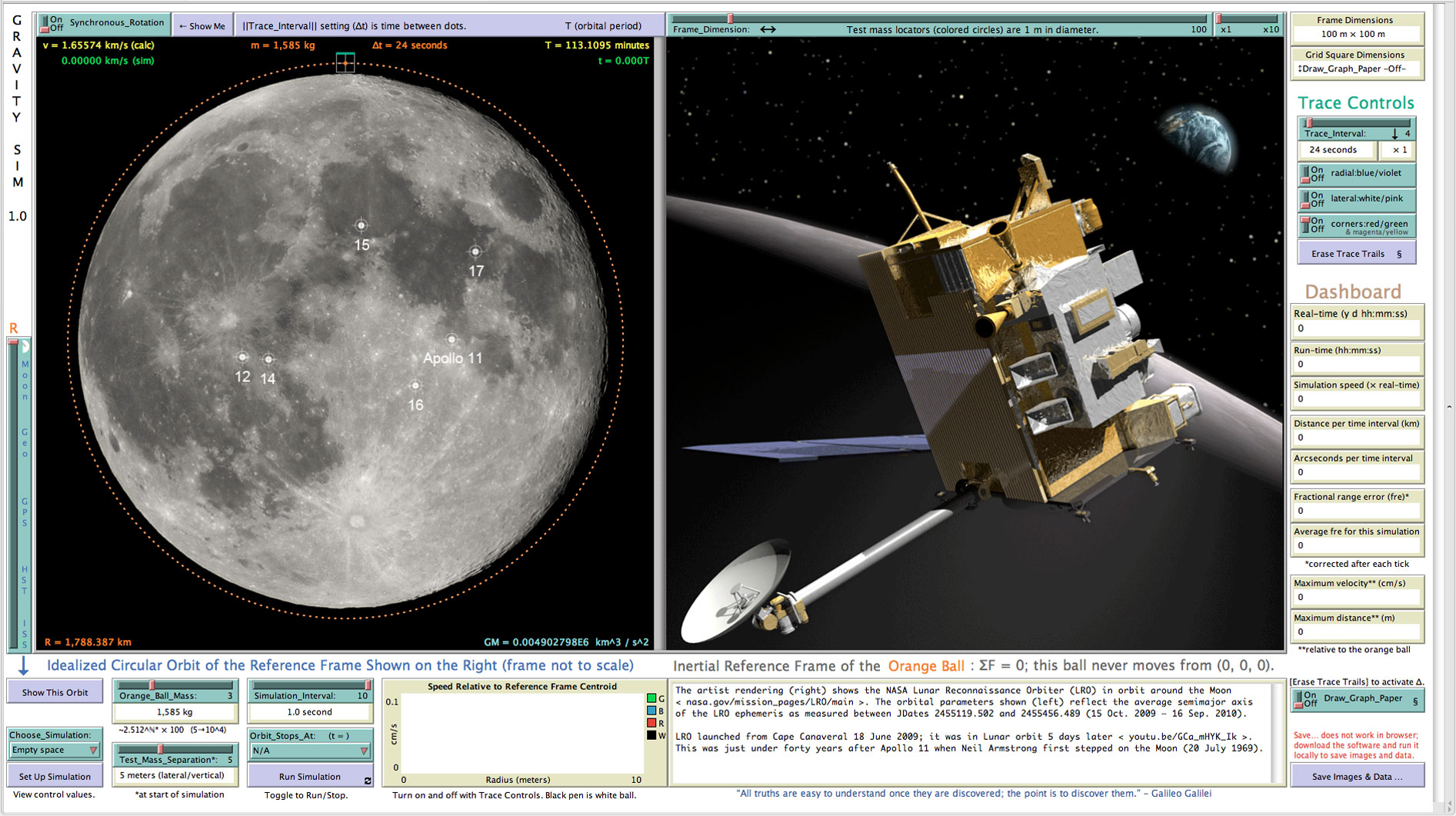# Gravity Sim screenshots   ( Netlogo environment )

## (1) Demonstration of orbiting gyroscope dynamics.This also shows the distinction between an ‘inertial’ orbit and an orbit with synchronous rotation.*

* (The rotation of an orbiting body on its axis in the same amount of time as it takes to complete a full orbit, with the result that the same face is always turned toward the body it is orbiting.)## (2)  Set up Simulation   for Radial free fall simulation.## Schematic of default radial free fall simulation highlighting the nature of lateral tidal forces.## (3) Default Radial free fall simulation (in progress).All Gravity Sim simulations are produced in a very simple way using the Euler method. Because the simulated reference-frame orbit is an idealized perfect circle (e = 0), intrinsic local error (error per step) is known, so the resulting minute position error of the reference-frame centroid can be corrected after each step. Consequently, the global error (error at a given time) in the position of the reference frame is always zero, which implies that the calculated accelerations on the test masses are equally accurate throughout a simulation. Because of the foregoing methodology, the simulated dynamical behavior of the test masses over time (right frame) is extremely accurate. Gravity Sim employs double-precision (64-bit) floating point numbers as defined in the IEEE 754 standard (binary64), which allows for approximately 16 decimal digits of calculation precision. If it is not legible on your screen, the message in orange at the bottom of the right frame (4, 5, 6) states: “Interaction is exclusively with the mass of the Earth and the orange ball [at the reference-frame centroid]; no interactions occur between the eight 1-gram test masses.”

## (4) Default Circular orbit simulation (in progress).## (6) Match the image simulation (in progress).## (7) Empty space simulation (in progress) — the identical simulation code for all simulations producing Keplerian elliptical orbits.## (8) Splash screen of commemorative Lunar Reconnaissance Orbiter (LRO) ephemeris, also showing landing sites of Apollo missions.